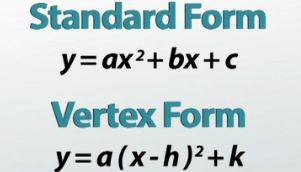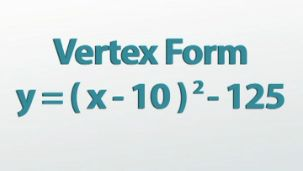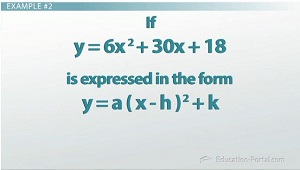# Completing the Square Practice Problems

An error occurred trying to load this video.

Try refreshing the page, or contact customer support.

Coming up next: How to Solve a Quadratic Equation by Factoring

### You're on a roll. Keep up the good work!

Replay
Your next lesson will play in 10 seconds
• 0:04 Completing the Square
• 0:52 Example #1
• 3:36 Example #2
• 6:54 Lesson Summary

Want to watch this again later?

Timeline
Autoplay
Autoplay
Speed

#### Recommended Lessons and Courses for You

Lesson Transcript
Instructor: Luke Winspur

Luke has taught high school algebra and geometry, college calculus, and has a master's degree in education.

Completing the square is one of the most confusing things you'll be asked to do in an algebra class. Once you get the general idea, it's best to get in there and actually do a few practice problems to make sure you understand the process. Do that here!

## Completing the Square

Completing the square is one of the most difficult things you'll be asked to do in an Algebra class. Not only does it require you to learn a bunch of new skills but you'll also need to remember a lot of older ones as well. Before we dive right into some practice problems, let's quickly review the basics.

Completing the square turns a quadratic equation in standard form into one in vertex form. A trinomial in standard form (y = ax2 + bx + c) can be factored into a perfect square binomial as long as the c-value on the end is equal to (b/2)2. If your trinomial's c-value isn't naturally equal to this exact right amount, you can 'complete the square' by performing operations on both sides of the equation to force the c-value into being exactly what you want it to be.## Example #1

So what does this look like in practice? Let's try changing this standard form equation (y = x2 - 20x - 25) into vertex form by completing the square. That requires us to turn this trinomial into a perfect square binomial. For this to work, the c-value of the trinomial needs to be the exact right number: (b/2)2. Let's check to see if this is already the right value. In this case, our b-value is -20, so -20/2 = -10 and (-10)2 = 100, so we're not in good shape yet. Right now our c-value is -25 but we need it to be 100. Luckily this is an equation, which means we can add or subtract something from both sides of the equals sign in order to change the c-value into exactly what we want it to be.

In this case, changing -25 into 100 means that we'll have to add 125 to both sides of the equation, leaving it like this: y + 125 = x2 - 20x + 100. At this point, as long as we haven't made a mistake, we can now factor the trinomial. Factoring requires us to look for two numbers that add up to the middle term, -20, and multiply to the constant on the end, 100. Quickly looking through the factors of 100, and then finding a pair of them that adds up to -20. So, -10 and -10 are our two winners, which means I can factor the trinomial on the right as (x - 10)(x -10). Which means the new equation will look like this: y + 125 = (x - 10)(x -10). Because the x - 10s are the same, I can combine them into one and write it as a square binomial, (x - 10)2, leaving our equation like this: y + 125 = (x - 10)2. We've now completed the square! All that's left to do is move the 125 over to the other side in order for the quadratic to be in vertex form. That makes y = (x - 10)2 - 125 the same equation as before, just expressed differently, and it's our final answer.As a note: Completing the square is actually often used to solve quadratic equations as well. If instead, this problem had not said complete the square, but solve, and instead of y we had a zero, we would have gotten an equation that looks like this: 0 = (x - 10)2 -125. Now we can simply use inverse operations to solve for x by undoing the 125, undoing the square with a square root and undoing -1 with a +1 to get our answer, like this: 1 +/- the square root of 125 = x. This is often what you'll use completing the square for - a way to solve a quadratic equation that's not factoring or the quadratic formula.

Although that may have seemed like a pretty long process, it was actually a straightforward example. Although the other problem we'll do during this lesson will basically be the same thing, it will have a few complexities that make it a little bit trickier.

## Example #2

First off, the directions are going to be a little bit different: If y = 6x2 + 30x + 18 is expressed in the form y = a(x - h)2 + k, what is the value of k? Different words, but same idea. Now though, once we've changed it to vertex form, the answer is simply the k-value and not the entire equation. But that's fine, same thing.To unlock this lesson you must be a Study.com Member.

### Register to view this lesson

Are you a student or a teacher?

### Unlock Your Education

#### See for yourself why 30 million people use Study.com

##### Become a Study.com member and start learning now.
Back
What teachers are saying about Study.com

### Earning College Credit

Did you know… We have over 160 college courses that prepare you to earn credit by exam that is accepted by over 1,500 colleges and universities. You can test out of the first two years of college and save thousands off your degree. Anyone can earn credit-by-exam regardless of age or education level.